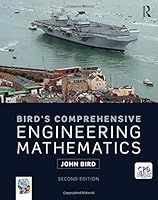# Bird’s Comprehensive Engineering Mathematics, 2nd Edition0 Reviews
2018-06-14
1226 pages

## Book Description

Studying engineering, whether it is mechanical, electrical or civil, relies heavily on an understanding of mathematics. This textbook clearly demonstrates the relevance of mathematical and shows how to apply them in real-life engineering problems.

It deliberately starts at an elementary level so that students who are starting from a low knowledge base will be able to quickly get up to the level required. Students who have not studied mathematics for some time will find this an excellent refresher.

Each chapter starts with the basics before gently increasing in complexity. A full outline of essential definitions, formulae, laws and procedures is presented, before real world practical situations and problem solving demonstrate how the theory is applied.

Focusing on learning through practice, it contains simple explanations, supported by 1600 worked problems and over 3600 further problems contained within 384 exercises throughout the text. In addition, 35 Revision tests together with 9 Multiple-choice tests are included at regular intervals for further strengthening of knowledge.

An interactive companion website provides material for students and lecturers, including detailed solutions to all 3600 further problems.

Section A Number and algebra
Chapter 1 Basic arithmetic
Chapter 2 Fractions
Chapter 3 Decimals
Chapter 4 Using a calculator
Chapter 5 Percentages
Chapter 6 Ratio and proportion
Chapter 7 Powers, roots and laws of indices
Chapter 8 , prefixes and engineering notation
Chapter 9 Basic algebra
Chapter 10 Further algebra
Chapter 11 Solving simple equations
Chapter 12 Transposing formulae
Chapter 13 Solving simultaneous equations
Chapter 15 Logarithms
Chapter 16 Exponential functions
Chapter 17 Inequalities
Section B Further number and algebra
Chapter 18 Polynomial division and the factor and remainder theorems
Chapter 19 Partial fractions
Chapter 20 Number sequences
Chapter 21 The binomial series
Chapter 22 Maclaurin’s series
Chapter 23 Solving equations by iterative methods
Chapter 24 Hyperbolic functions
Chapter 25 Binary, octal and hexadecimal numbers
Chapter 26 Boolean algebra and logic circuits
Section C Areas and volumes
Chapter 27 Areas of common shapes
Chapter 28 The circle and its properties
Chapter 29 Volumes and surface areas of common solids
Chapter 30 Irregular areas and volumes, and mean values
Section D
Chapter 31 Straight line graphs
Chapter 32 Graphs reducing non-linear laws to linear form
Chapter 33 Graphs with logarithmic scales
Chapter 34 Polar curves
Chapter 35 Graphical solution of equations
Chapter 36 Functions and their curves
Section E Geometry and trigonometry
Chapter 37 Angles and triangles
Chapter 38 Introduction to trigonometry
Chapter 39 Trigonometric waveforms
Chapter 40 Cartesian and polar co-ordinates
Chapter 41 Non-right-angled triangles and some practical applications
Chapter 42 Trigonometric identities and equations
Chapter 43 The relationship between trigonometric and hyperbolic functions
Chapter 44 Compound angles
Section F Complex numbers
Chapter 45 Complex numbers
Chapter 46 De Moivre’s theorem
Section G Matrices and determinants
Chapter 47 The theory of matrices and determinants
Chapter 48 Applications of matrices and determinants
Section H Vector geometry
Chapter 49 Vectors
Chapter 50 Methods of adding alternating waveforms
Chapter 51 Scalar and vector products
Section I Differential
Chapter 52 Introduction to differentiation
Chapter 53 Methods of differentiation
Chapter 54 Some applications of differentiation
Chapter 55 Differentiation of parametric equations
Chapter 56 Differentiation of implicit functions
Chapter 57 Logarithmic differentiation
Chapter 58 Differentiation of hyperbolic functions
Chapter 59 Differentiation of inverse trigonometric and hyperbolic functions
Chapter 60 Partial differentiation
Chapter 61 Total differential, rates of change and small changes
Chapter 62 Maxima, minima and saddle points for functions of two variables
Section J Integral calculus
Chapter 63 Standard
Chapter 64 Integration using algebraic substitutions
Chapter 65 Integration using trigonometric and hyperbolic substitutions
Chapter 66 Integration using partial fractions
Chapter 67 The t = tan ./2 substitution
Chapter 68 Integration by parts
Chapter 69 Reduction formulae
Chapter 70 Double and triple integrals
Chapter 71 Numerical integration
Chapter 72 Areas under and between curves
Chapter 73 Mean and root mean square values
Chapter 74 Volumes of solids of revolution
Chapter 75 Centroids of simple shapes
Chapter 76 Second moments of area
Section K Differential equations
Chapter 77 Solution of first-order differential equations by separation of variables
Chapter 78 Homogeneous first-order differential equations
Chapter 79 Linear first-order differential equations
Chapter 80 Numerical methods for first-order differential equations
Chapter 81 Second-order differential equations of the form a d2y dx2 +b dy dx +cy=0
Chapter 82 Second-order differential equations of the form a d2y dx2 +b dy dx +cy=f (x
Chapter 83 Power series methods of solving ordinary differential equations
Chapter 84 An introduction to partial differential equations
Section L Statistics and probability
Chapter 85 Presentation of statistical data
Chapter 86 Mean, median, mode and standard deviation
Chapter 87 Probability
Chapter 88 The binomial and Poisson distributions
Chapter 89 The normal
Chapter 90 Linear correlation
Chapter 91 Linear regression
Chapter 92 Sampling and estimation theories
Chapter 93 Significance testing
Chapter 94 Chi-square and distribution-free tests
Section M Laplace transforms
Chapter 95 Introduction to Laplace transforms
Chapter 96 Properties of Laplace transforms
Chapter 97 Inverse Laplace transforms
Chapter 98 The Laplace transform of the Heaviside function
Chapter 99 The solution of differential equations using Laplace transforms
Chapter 100 The solution of simultaneous differential equations using Laplace transforms
Section N Fourier series
Chapter 101 Fourier series for periodic functions of period 2p
Chapter 102 Fourier series for a non-periodic function over range 2p
Chapter 103 Even and odd functions and half-range Fourier series
Chapter 104 Fourier series over any range
Chapter 105 A numerical method of harmonic analysis
Chapter 106 The complex or exponential form of a Fourier series
Section O Z-transforms
Chapter 107 An introduction to z-transforms

## Book Details

• Title: Bird’s Comprehensive Engineering Mathematics, 2nd Edition
• Author:
• Length: 1226 pages
• Edition: 2
• Language: English
• Publisher:
• Publication Date: 2018-06-14
• ISBN-10: 0815378149
• ISBN-13: 9780815378143